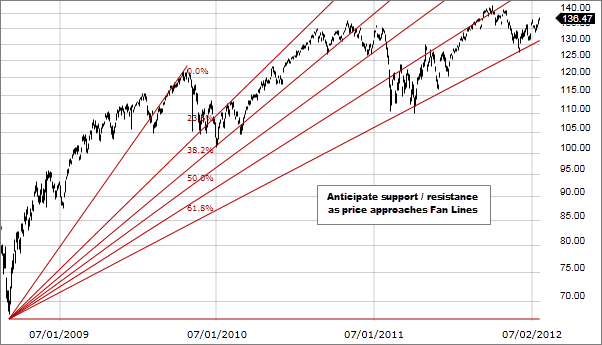# Fibonacci: Fans

No comment 691 views
5 / 5 ( 1votes )

# Fibonacci: FansFibonacci: Fans

Fibonacci Fans that more can be interpreted as Fibonacci fan or fan-like shape than Fibonacci fans. Fibonacci Fans are based on the same principles as Fibonacci Retracement but have more in common with Gann lines and Speed ​​Resistance Lines (SRL). Just like in Fibonacci Retracement, Fibonacci Fans are based on the mathematical relationship between numbers in the Fibonacci sequence. However, Fibonacci Fans illustrate trend line rather than horizontal support and resistance line.

The Fibonacci fan line is determined by applying the Fibonacci ratios to significant high and low levels that mark the start of a trend. The Fibonacci ratio is determined by dividing the numbers in sequence with the other numbers that follow.

The first ratio of 61.8%, which is the opposite of the “golden ratio” or “gold mean” (ie the opposite of 1.61%), is found by dividing the numbers in sequences by numbers directly following them. For example: 8 ÷ 13 = 0.6153, 34 ÷ 55 = 0.6182 and 55 ÷ 89 = 0.6179.
The ratio of 38.2% is found by dividing the number in sequence by the number following the two places to the right. For example: 8 ÷ 21 = 0.3809, 21 ÷ 55 = 0.3818 and 55 ÷ 144 = 0.3819. It is also found by subtracting 0.618 from 1 (1 – 0.618 = 0.328).
And the 23.6% ratio is found by dividing the numbers in sequence with the numbers following the three places to the right. For example: 8 ÷ 34 = 0.2352, 21 ÷ 89 = 0.2359 and 34 ÷ 144 = 0.2361. It is also found by subtracting 0.382 from 0.618 (0.618 – 0.382 = 0.236).
In addition to these three ratios, some traders also use a 50% ratio. While this is not the Fibonacci ratio, it appears to be a significant level that price movements must adhere to.
When the beginning of a trend has been identified the range from high to significant low marks the start of the trend divided by the Fibonacci ratio and these lines are drawn through these levels, starting from the high if the current trend is a downtrend. or a low if the current trend is an ascending trend.

Like other support and resistance indicators, Fibonacci Fans are based on the principle that the market will retrace some of the previous moves before proceeding towards the trend. This is based on the fact that financial market trends are not in a straight line. Instead the trend is interrupted by retracements that tend not to exceed the previous wave.Forex Signal 30 is the best forex system since 2009 and has been used by thousands of traders from around the world to generate profit in forex trading. This system is created by our team of Brilliant Forex Signal Team, this system is made as simple as possible for beginner and professional traders.